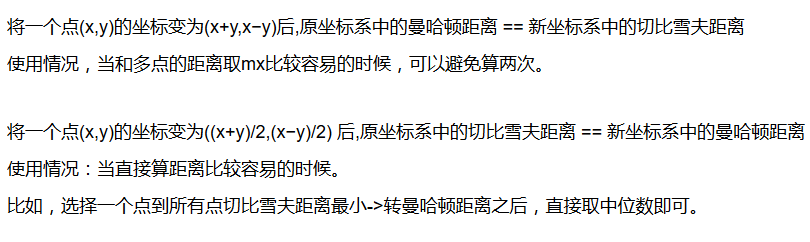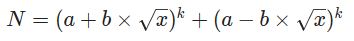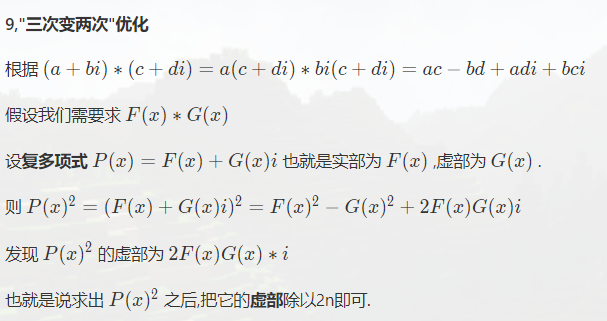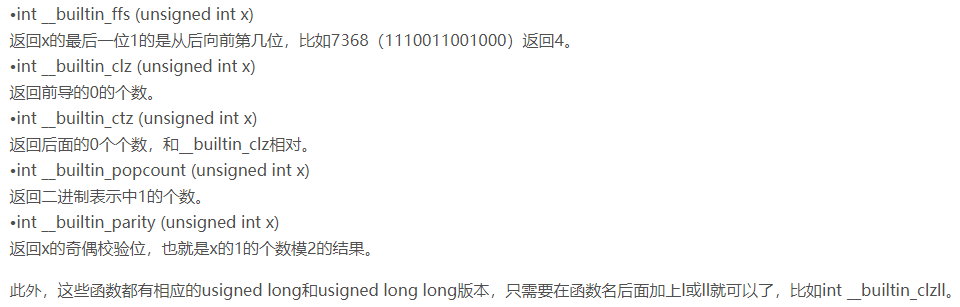# 奇技淫巧

## 0.如果不是秒切的话，解决问题的一般思路：

1.退而求其次：如果数据小、没有某个限制怎么办？（可以通过暴力分得到提示）

2.找到矛盾问题的根源，就是算法升级的关键，如果有了这个限制，怎么处理？

3.集中处理这个矛盾

## 1.快读：

void read(int &x)
{
char ch;bool flag=false;
while(!isdigit(ch=getchar()))
(ch=='-')&&(flag=true);
for(x=num;isdigit(ch=getchar());x=x*10+num);
(flag)&&(x=-x);
}

## 2.取模优化：

inline int mod(int x)
{
return x-p*(x/p);
}

## 3.线段树define操作

struct node{
int l,r;
int mx,hx;
#define ch(x) t[x].ch
#define mx(x) t[x].mx
#define hx(x) t[x].hx
#define hc(x) t[x].hch
#define l(x) t[x].l
#define r(x) t[x].r
}t[4*N];

## 8.字符读入避免空格

ztb法：char s[] scanf("%s",s+1) 利用字符串自动剔除前面空格，并且读到下一个空格位置。

chen_zhe法：char c scanf("%s",&c) 敢情这也可以。。。。

## 9.Hungary匈牙利算法每次memset的小优化（默认左部点出发dfs）

①一般情况下，我们是每次找到一个右部点，vis[y]=1，之后每次memset一下vis

②但是，有的时候，右部点很多，memset一下，nleft*nright 就挂了。

③我们也可以用一个栈sta[]，收集右部点vis的点，用弹栈并归零处理vis。

（2018.9.8  update:其实根本可以不用每次尝试清空什么vis！！！

int vis[N],pre[N];
int id;
bool dfs(int x){
for(int i=hd[x];i;i=e[i].nxt){
int y=e[i].to;
if(vis[y]!=id){
vis[y]=id;
if(!pre[y]||dfs(pre[y])){
pre[y]=x;
return true;
}
}
}
return false;
}
for(int i=1;i<=mx;i++){
id++;
if(dfs(i)) ans++;
}

## 10.多个点的lca

dfs序最小点和dfs序最大点取lca即可。

## 11.区间维护最小值，支持删除，插入

1.multiset

2.差的最小值呢？两个set，第二个set维护数值set相邻两个的差值。插入删除用前驱后继处理。

## 13.曼哈顿距离转切比雪夫距离

(x,y) -> (x-y,x+y)重新设置点的坐标。

(x1,y1),(x2,y2) -> (x1+y1,x1-y1), (x2+y2,x2-y2)

## 15.一点反演技巧

(i,j)=1 时，等价于：∑d d|i&&d|j u(d) =1

(i,j)!=1时，等价于：∑d d|i&&d|j u(d) =0

i，j互质的时候，公约数只有1，u(1)=1

i，j不互质的时候，公约数就是i，j最大公约数的约数。

。。。

## 16数学期望dp小结

1.多个终点一个起点，就f[i]表示，从i到终点的期望步数，f[s]即为答案

2.多个起点一个终点，就f[i]表示，从起点到i的期望步数，f[t]即为答案

3.与终点无关的树形期望dp，通常往子树对i，父亲对i影响考虑，和一般的树形dp类似。

## 17.曼哈顿距离和切比雪夫距离转化## 19.扩展欧拉定理：

$b^c\space mod p=b^{c\space mod p+\phi(p)}mod p (c>\phi(p))$

p,b可以不互质

S=|x1y2-x2y1|/2

## 22.无理数的高精度处理

（适用于($(a+b\times\sqrt{x})^k$）：

1.保留“a+b*根号”的形式：构造有序数对：(a,b)，即可定义乘法。

2.精度问题：令## 24.十进制快速幂（专治高精快速幂）

y要循环$log_{10} y$次。

（由于复杂度在低精度下是一样的，所以除了高精快速幂，并没有什么卵用）

## 26.HASH判断循环节

hash(sj)*p^leni+hash(si)=hash(si)*p^lenj+hash(sj)

## 28.所有取值的前k最值问题

1.起初把每个点的最优解找出来，带着点的id，放进堆里面。

2.从堆里取出最优解。

3.把和这个id有关的次优解找出来，放进堆里。

[NOI2010]超级钢琴

## 30.-Wall -W

#pragma GCC ("-W1,--stack=128000000)手动开栈。

## 31.对于一些序列两次取值的问题（两次取值位置不相交）

ans=max(ans,f[i]+g[i])

## 33.NOI Linux编译

1.进入Linux虚拟机

2.桌面新建文件。打开。

3.复制代码进去。

4.重命名为my.cpp（后缀为cpp）

5.Ctrl+Alt+T 进入终端

6.输入cd Desktop进入桌面界面（忘了文件名，输入ls Desktop 显示桌面的所有文件）

7.输入g++ my.cpp -o my.exe

（有需要的话，后面加上-std=c++11 -Wall -O2）

8.如果回车之后，没有其他信息（没有error），那么编译成功。

9.接下来输入./my.exe （注意之间没有空格）表示进入这个exe

（按动↑可以寻找上面进行过的命令）

## 35.int a=floor((double)b+0.5)

（注意，负数的时候，强转int是向中取整，floor是向下取整，有所不同。看情况决定）

for(reg i=0;i<=m;++i){
b[i].x/=n;
int pp=b[i].x+0.5;
printf("%d ",pp);
}

for(reg i=0;i<=m;++i){
b[i].x/=n;
printf("%.0lf ",fabs(b[i].x));
}

还有一个东西叫round(x)

(int)y=round((double)x)

## 36.FFT三次变两次：

P(x)^2虚数部分/2即可。节省一次DFT## 38.堆优化dij的一个小剪枝

1.不必每次把所有的出边更新到的都加入到堆里

2.还可以用num记录已经确定的点的个数，如果num=0直接return

## 39.关于平均值的二分

fzyzojP1635 -- 平均值

2.11考试

## 42.异或下线性方程组的自由元个数：

（一般的线性方程组也是这样求，只不过xor下的自由元用的比较多）

## 43.一个通用技巧是：

f范围宽松好统计，g范围严格难统计但是和答案有直接关系，

luoguP4841 城市规划

bzoj4671: 异或图——斯特林反演

[学习笔记]拉格朗日插值

## 46.一种生成函数还原序列的方法

①如果一个生成函数的次方的话，就是组合意义小球放盒子了，O(1)有公式，LUCAS求组合数

②否则考虑能不能把其中一个的生成函数变成有限项，使得项数在O(n)以内

PK LCT

## 49.取Ln

1.有标号图计数，F表示所有情况，G表示连通情况。则：G=Ln(F)

luoguP4841 城市规划

COGS 2353 2355 2356 2358 有标号的DAG计数

2.把乘法变成加法，

①多项式降次

luoguP4705 玩游戏

②把答案大的变小，仍然满足单调性，所以取最小值方案还是不变的。

[BJOI2019]奥术神杖 取Ln直接就变成0/1分数规划了

3.碰见Ln(f)很麻烦？

4.求积分时候，处理分式分母

## 52.## 53.真·奇技淫巧

O(p)预处理，O(1)任意底数，任意质数的快速幂

$a^b=G^{log_G{a^b}}=G^{b\times log_Ga}$

## 55.编号区间点集，信息可以合并？

Max Mex 51nod1766

## 56.不能立刻决定球的颜色？

UOJ#449. 【集训队作业2018】喂鸽子

## 57.IDFT的trick

UOJ#424. 【集训队作业2018】count

## 58.__builtin## 61.bitset与字符串匹配？

[国家集训队]JZPSTR

xi=x%Mi+1Mi

2.

posted @ 2018-05-13 10:48  *Miracle*  阅读(1621)  评论(0编辑  收藏  举报